Courses

## 20 Questions MCQ Test Mathematics (Maths) Class 6 | Olympiad Test: Algebra

Description
This mock test of Olympiad Test: Algebra for Class 6 helps you for every Class 6 entrance exam. This contains 20 Multiple Choice Questions for Class 6 Olympiad Test: Algebra (mcq) to study with solutions a complete question bank. The solved questions answers in this Olympiad Test: Algebra quiz give you a good mix of easy questions and tough questions. Class 6 students definitely take this Olympiad Test: Algebra exercise for a better result in the exam. You can find other Olympiad Test: Algebra extra questions, long questions & short questions for Class 6 on EduRev as well by searching above.
QUESTION: 1

### Which of the following are used to represent unknown quantities in algebra?

Solution:

In algebra, unknown quantities are represented by lower case letters.

QUESTION: 2

### What do literals usually represent?

Solution:

Literals usually represent variables.

QUESTION: 3

### The rule, which gives the number of matchsticks required to make the matchstick pattern L, is

Solution: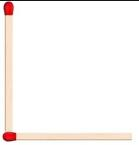from the figure it is confirmed that we need 2 matchstick minium for a 'L' shape. so matchsticks will be multiple of 2. so, we need 2n matchsticks

QUESTION: 4

What is the equation for the statement 'half of a number added to 10 is 15'?

Solution:
QUESTION: 5

Write an equation for the statement 'thrice the length of a room is 340 metres'.

Solution:
QUESTION: 6

What is the method of finding a solution by trying out various values for the variable called?

Solution:
QUESTION: 7

A Find the value of 'a' that satisfies the equation 6a = −144.

Solution:

6a = -144

a = -144/6

a = -24

QUESTION: 8

Choose a value of 'a' that satisfies the equation 6a = -30.

Solution:

6a = −30 ⇒ a = -30/6 = -5

QUESTION: 9

What is the value of x if 3x/4 +8 =17?

Solution: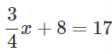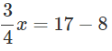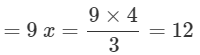QUESTION: 10

For what value of 'a' is 6(2a-1)+8 = 14 true?

Solution:
QUESTION: 11

Determine the value of x given 6x−1 = 2x+9.

Solution:
QUESTION: 12

Ramu's father is thrice as old as Ramu. If father's age is 45 years, how old is Ramu?

Solution:
QUESTION: 13

A number is multiplied by 6 and 12 is added to the product. The result is 84. What is the number?

Solution:

Let the number be x. It is multiplied by
6 ⇒ 6x 12 is added to the product
⇒ 6x+12
The result is 84 ⇒ 6x+12 = 84 ⇒ 6x = 72
∴ x = 12
(By trial and error method).

QUESTION: 14

Find the length of a side of an equilateral triangular garden whose perimeter is 66 m.

Solution:

Let the side of an equilateral triangular garden be x m.
So, 3x = 66 ⇒ x = 22m

QUESTION: 15

In the given figure, what are the magnitudes of the angles shown?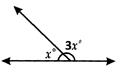Solution:

From the figure,x + 3x = 180 (straight angle)
⇒ 4x∘ =180
⇒x = 45
∴ 3x∘ = 3×45∘ = 135

QUESTION: 16

The length of a rectangle is twice its breadth. If its area is 72 sq. m, find the breadth of the rectangle.

Solution:

Let the breadth of the rectangle be b units. The length is twice its breadth.

⇒ Its length = 2b units

∴  Area = b × 2b sq units

⇒2×b×b = 72 b × b=  72/2 ⇒b × b = 36 = 6 × 6

⇒ b = 6m

QUESTION: 17

Which of the following is the perimeter of a regular hexagon of side 's' units?

Solution:

A regular hexagon has 6 equal sides. Hence perimeter = 6s units.

QUESTION: 18

Which of the following does 2n  -1 represent?

Solution:

2n - 1 is 1 subtracted from the product of 2 and n.

QUESTION: 19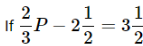what is the value of p?

Solution: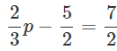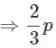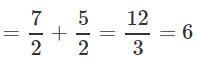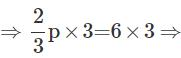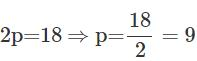QUESTION: 20

In a piggy bank the number of 25 paise coins is five times the number of 50 paise coins. If there are 120 coins, find the amount in the bank.

Solution:

Let the number of 50 paise coins be x.
⇒ 25 paise coins = 5x
Given that total coins = 120
⇒ x + 5x = 120
⇒ 6x = 120
⇒ x = 120/6 = 20
∴ 50 paise coins = 20
⇒ Amount = 20×50 p = Rs. 10
Value of 25 paise coins = 25p × 5 × 20
= 25p × 100 = Rs. 25
Total amount Rs. (25 + 10) = Rs. 35